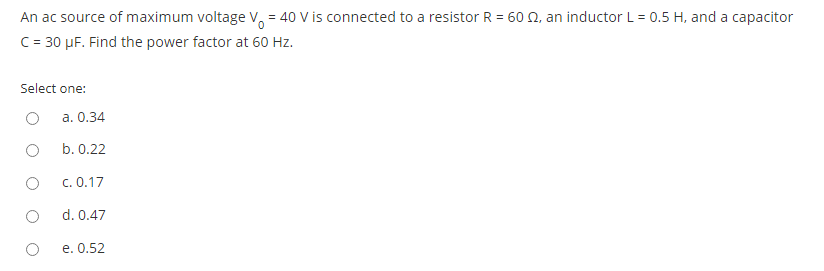# Question An ac source of maximum voltage V. = 40 V is connected to a resistor R = 60 12, an inductor L = 0.5 H, and a capacitor C = 30 uF. Find the power factor at 60 Hz. Select one: a. 0.34 b. 0.22 O C. 0.17 d. 0.47 O e. 0.52